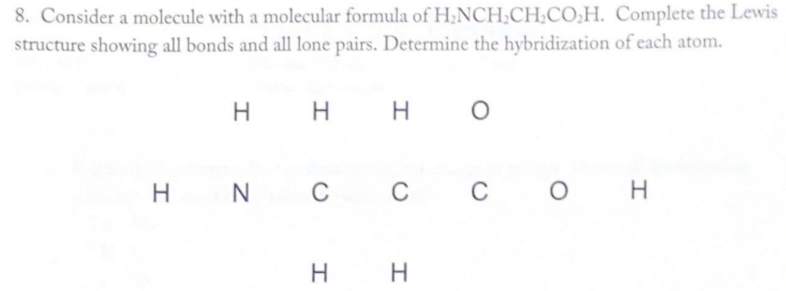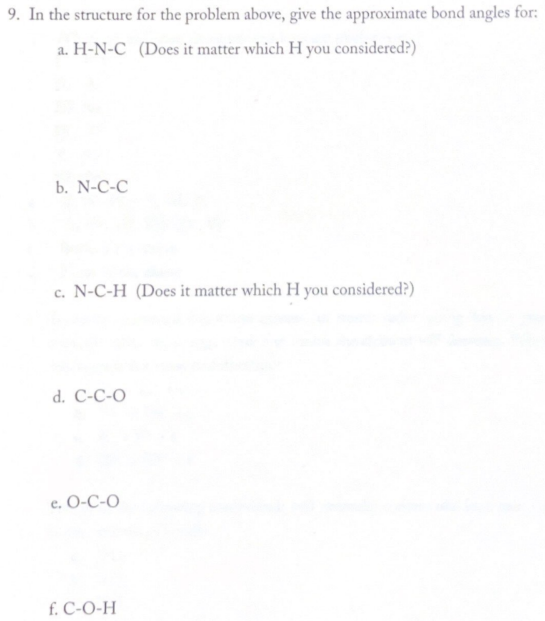# 8. Consider a molecule with a molecular formula of H2NCH2CH2CO2H. Complete the Lewis structure showing all bonds and all lone pairs. Determine the hybridization of each atom. 9. In the structure for the problem above, give the approximate bond angles for: a. H-N-C (Odes it matter which H you considered?) b. N -C-C c. N-C-H (Does it matter which H you considered?) d. C-C-O e. O-C-C f. C-O-H